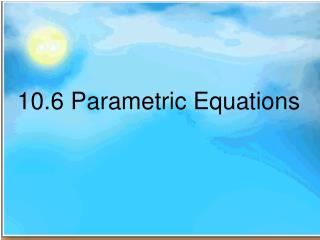DownloadDownload Presentation10.6 Parametric Equations

# 10.6 Parametric Equations

Download Presentation## 10.6 Parametric Equations

- - - - - - - - - - - - - - - - - - - - - - - - - - - E N D - - - - - - - - - - - - - - - - - - - - - - - - - - -
##### Presentation Transcript

1. 10.6 Parametric Equations

2. Definition of Parametric Equations parametric equation is a method of defining a relation using parameters. A simple kinematic1 example is when one uses a time parameter to determine the position, velocity, and other information about a body in motion. 1. Kinematic.The branch of mechanics that studies the motion of a body or a system of bodies without consideration given to its mass or the forces acting on it. http://en.wikipedia.org/wiki/Parametric_equation

3. We will not be finding parametric equations, in this example. In this class we will graph parametric equation also eliminating the parameter. Given the equation With respect to time Rectangular equation Parametric Equations The parametric equations have to be Continuous

4. Both Equations graph the same. The Parametric states how fast to graph Given the equation With respect to time Rectangular equation Parametric Equations

5. Graphing Parameter equations Parameter equations

6. Graphing Parameter equations Parameter equations

7. Change to Rectangular Equation Parameter equations to Rectangular equation

8. Change from Parametric to Rectangular Parametric Equations

9. Change from Parametric to Rectangular Parametric Equations

10. Change from Parametric to Rectangular Parametric Equations

11. Change from Parametric to Rectangular Parametric Equations

12. Change from Parametric to Rectangular Parametric Equations

13. Change from Parametric to Rectangular Parametric Equations

14. Change from Parametric to Rectangular x = h + a Cosθ y = k + b Sinθ

15. Change from Parametric to Rectangular x = h + a Cosθ y = k + b Sinθ

16. What would the parametric equation be for a circle with center at (3,2) and radius of 4 (x – h)2 + (y – k)2 = r2 In a circle a = b = 4

17. Homework Page 747 – 749 # 7, 14, 21, 28, 32, 41, 48

18. Homework Page 747 – 749 # 5, 10, 15, 20, 25, 30, 35, 40